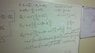# Ray Deflection of a Prism

Axis001

## Homework Statement

The problem I am having troubles with is proving the deflection of a ray by a prism can be represented by a specific equation : θd = θ - α + arcsin(sin(α)√(n2 - sin2(α)) - sin(α)cos(α)). I have derived another form of the deflection equation for a prism and I am attempting to move it into the form requested but I am getting stuck on some trigonometry.

## Homework Equations

α is the apex angle of the prism and θ is the angle of incidence on the prism.

## The Attempt at a Solution

See attachments.

#### Attachments

•2012-08-25_21-16-59_758.jpg
22.5 KB · Views: 519
Last edited:

Homework Helper
Gold Member
Hello, Axis001. Could you please define the symbols, especially the meaning of θ and α?

Axis001
My apologies not sure how I overlooked defining my variables. α is the apex angle of the prism and θ is the angle of incidence on the prism.

Homework Helper
Gold Member
Is your attachment showing your own work? Are you trying to find a mistake in the attachment?

I'm guessing that θ1 and θ'1 are the angles of incidence and refraction, respectively, at the first refraction; whereas, θ'2 and θ2 are the angles of incidence and refraction, respectively, at the second refraction. (That is, the primes denote angles inside the prism.)

Axis001
The work in the attachment is mine and your assumption of origin of angles of refraction is correct. I have simplified the equation for the displacement angle I originally found θd = θ - α + arcsin(nsin(α - arcsin((1/n)sin(θ))) into terms of simply θ, n, and α. The last step shown in the attachment is where I am currently stuck. I currently have the displacement of the prism at θd = θ - α + arcsin(nsin[sin(α)√(n2 - sin2(α)) - sin(θ)cos(α))] and I simple cannot figure out how to reduce it to the required form of θd = θ - α + arcsin(sin(α)√(n2 - sin2(α)) - sin(θ)cos(α)). I have tried every single trigonometric identity I can think of and none of them give the reduced equation I am looking for.

Last edited:
Homework Helper
Gold Member
In the attachment, the third to last equation looks ok. However, in going to the final expression there appears to be two mistakes:
(1) the cosine factor is not quite simplified correctly (going from the next to last line to the last line)
(2) there’s a place where you took no/n1 to be n instead of 1/n (going from the third to last line to the next to last line)

Axis001

(2) there’s a place where you took no/n1 to be n instead of 1/n (going from the third to last line to the next to last line)

Ah yes I'm not sure how I missed.

(1) the cosine factor is not quite simplified correctly (going from the next to last line to the last line)

With the correction to the incorrectly stated index of refraction the correct reduction from the second to last line to the last line should be:

θd = θ - α + arcsin(nsin[sin(α)√(n2 - sin2(α)) - (1/n)sin(θ)cos(α)])

Which leaves me with the problem of the nsin(...) term in the equation. The required equation θd = θ - α + arcsin(sin(α)√(n2 - sin2(α)) - sin(θ)cos(α)) which is just the equation I currently have with the nsin(...) term eliminated from it. I have tried using sum property for sines again to see if it generates the required equation but it just provides a useless and incredibly complicated new equation.

Homework Helper
Gold Member
Note that cos[sin-1(β)] = √(1- sin2[sin-1(β)]) = √(1-β2)

Axis001
Ok from "start" to current step with the corrections you helped me find this is what I have.

θd = θ - α + arcsin(nsin(α - arcsin((1/n)sin(θ)))
θd = θ - α + arcsin(nsin[sin(α)cos[arcsin((1/n)sin(θ))] - cos(α)sin[arcsin((1/n)sin(θ))])
θd = θ - α + arcsin(nsin[sin(α)sqrt[1 - (sin2(θ))/n2] - (1/n)cos(α)sin(θ))
θd = θ - α + arcsin(nsin[(1/n)sin(α)sqrt[n2 - sin2(θ)] - (1/n)cos(α)sin(θ))
θd = θ - α + arcsin[nsin[(1/n)[sin(α)sqrt[n2 - sin2(θ)] - cos(α)sin(θ)]]]

Homework Helper
Gold Member
Ok from "start" to current step with the corrections you helped me find this is what I have.

θd = θ - α + arcsin(nsin(α - arcsin((1/n)sin(θ)))
θd = θ - α + arcsin(nsin[sin(α)cos[arcsin((1/n)sin(θ))] - cos(α)sin[arcsin((1/n)sin(θ))])
θd = θ - α + arcsin(nsin[sin(α)sqrt[1 - (sin2(θ))/n2] - (1/n)cos(α)sin(θ))
θd = θ - α + arcsin(nsin[(1/n)sin(α)sqrt[n2 - sin2(θ)] - (1/n)cos(α)sin(θ))
θd = θ - α + arcsin[nsin[(1/n)[sin(α)sqrt[n2 - sin2(θ)] - cos(α)sin(θ)]]]

Oh, I overlooked another mistake which I highlighted in red above. Should those sin's be there?

Axis001
Oh, I overlooked another mistake which I highlighted in red above. Should those sin's be there?

Yes the nsin(...) terms should be there and it is what is giving me such a head ache since I just simply cannot get it out of the equation. A form of the deflection equation that I know is θd = θ - α + arcsin(nsin(α - arcsin((1/n)sin(θ))). It is easily derivable from applying Snell's Law at both "sides" of the prism and the expression of θd = θ1 + θ2 - α. Solving for θ2 into a expression of θ1 and α will result in the deflection equation I started this with but it results with a nsin(...) term I just can't seem to get out of my equations.

Homework Helper
Gold Member
Yes the nsin(...) terms should be there

Hmm. Which is correct:

sin(α - β) = sinαcosβ-cosαsinβ

or

sin(α - β) = sin[sinαcosβ-cosαsinβ]

Axis001I need to go to sleep. Thank you so much I really appreciate it.

Homework Helper
Gold MemberI need to go to sleep.

Me too. :zzz: